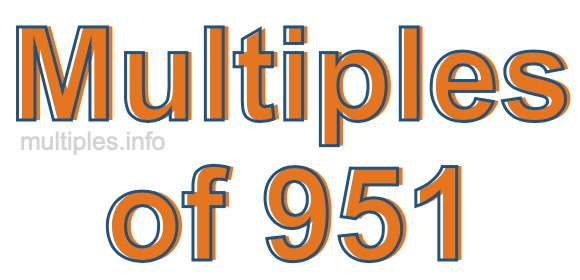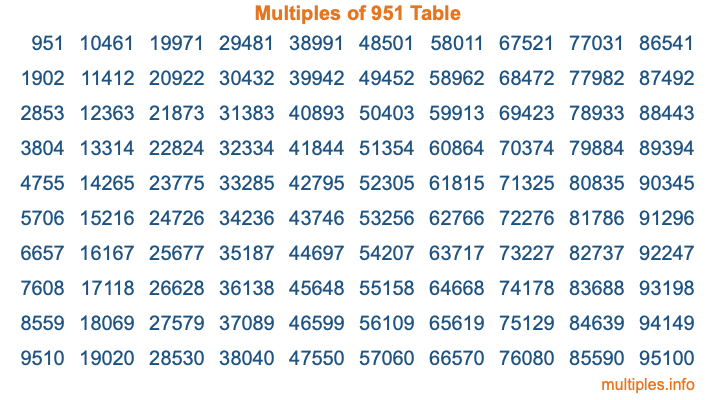Multiples of 951Welcome to the Multiples of 951 page. Here we will first teach you everything you will ever need to know about the multiples of 951, and then give you a study guide summary of everything we taught you to make sure you remember it all. Use this page to look up facts and learn information about the multiples of 951. This page will make you a multiples of nine hundred fifty-one expert!

Definition of Multiples of 951
Multiples of 951 are all the numbers that when divided by 951 equal an integer. Each of the multiples of 951 are called a multiple. A multiple of 951 is created by multiplying 951 by an integer.

Therefore, to create a list of multiples of 951, you start with 1 multiplied by 951, then 2 multiplied by 951, then 3 multiplied by 951, and so on for as long as you want. Thus, the list of the first five multiples of 951 is 951, 1902, 2853, 3804, and 4755. To see a larger list of multiples of 951, see the printable image of Multiples of 951 further down on this page. We also have a category where you can choose any nth multiple of 951.

Multiples of 951 Checker
The Multiples of 951 Checker below checks to see if any number of your choice is a multiple of 951. In other words, it checks to see if there is any number (integer) that when multiplied by 951 will equal your number. To do that, we divide your number by 951. If the the quotient is an integer, then your number is a multiple of 951.

Is  a multiple of 951?

Least Common Multiple of 951 and ...
A Least Common Multiple (LCM) is the lowest multiple that two or more numbers have in common. This is also called the smallest common multiple or lowest common multiple and is useful to know when you are adding our subtracting fractions. Enter one or more numbers below (951 is already entered) to find the LCM.

Check out our LCM Calculator if you need more details about the Least Common Multiple or if you need the LCM for different numbers for adding and subtraction fractions.

nth Multiple of 951
As we stated above, 951 is the first multiple of 951, 1902 is the second multiple of 951, 2853 is the third multiple of 951, and so on. Enter a number below to find the nth multiple of 951.

th multiple of 951

Multiples of 951 vs Factors of 951
951 is a multiple of 951 and a factor of 951, but that is where the similarities end. All postive multiples of 951 are 951 or greater than 951. All positive factors of 951 are 951 or less than 951.

Below is the beginning list of multiples of 951 and the factors of 951 so you can compare:

Multiples of 951: 951, 1902, 2853, 3804, 4755, etc.

Factors of 951: 1, 3, 317, 951

As you can see, the multiples of 951 are all the numbers that you can divide by 951 to get a whole number. The factors of 951, on the other hand, are all the whole numbers that you can multiply by another whole number to get 951.

It's also interesting to note that if a number (x) is a factor of 951, then 951 will also be a multiple of that number (x).

Multiples of 951 vs Divisors of 951
The divisors of 951 are all the integers that 951 can be divided by evenly. Below is a list of the divisors of 951.

Divisors of 951: 1, 3, 317, 951

The interesting thing to note here is that if you take any multiple of 951 and divide it by a divisor of 951, you will see that the quotient is an integer.

Multiples of 951 Table
Below is an image of the first 100 multiples of 951 in a table. The table is in chronological order, column by column. The first column has the first ten multiples of 951, the second column has the next ten multiples of 951, and so on.The Multiples of 951 Table is also referred to as the 951 Times Table or Times Table of 951. You are welcome to print out our table for your studies.

Negative Multiples of 951
Although not often discussed or needed in math, it is worth mentioning that you can make a list of negative multiples of 951 by multiplying 951 by -1, then by -2, then by -3, and so on, to get the following list of negative multiples of 951:

-951, -1902, -2853, -3804, -4755, etc.

Multiples of 951 Summary
Below is a summary of important Multiples of 951 facts that we have discussed on this page. To retain the knowledge on this page, we recommend that you read through the summary and explain to yourself or a study partner why they hold true.

There are an infinite number of multiples of 951.

A multiple of 951 divided by 951 will equal a whole number.

951 divided by a factor of 951 equals a divisor of 951.

The nth multiple of 951 is n times 951.

The largest factor of 951 is equal to the first positive multiple of 951.

951 is a multiple of every factor of 951.

951 is a multiple of 951.

A multiple of 951 divided by a divisor of 951 equals an integer.

951 divided by a divisor of 951 equals a factor of 951.

Any integer times 951 will equal a multiple of 951.

Multiples of a Number
Here you can get the multiples of another number, all with the same attention to detail as we did for multiples of 951 on this page.

Multiples of
Multiples of 952
Did you find our page about multiples of nine hundred fifty-one educational? Do you want more knowledge? Check out the multiples of the next number on our list!